# Hirota Solitons

## Hirota-mKdV Equation ut + 24|u|2ux + uxxx = 0

There are two equivalent forms for the Hirota-mKdV equation:
(1) In complex-variable notation, u = u1 + i u2 satisfies

ut + 24 uuux + uxxx =0

(2) In vector notation, u = (u1, u2) satisfies

ut + 24 uu ux + uxxx =0

### Travelling Wave Solution

Travelling waves are given by the special soliton solution

u(t,x)= (√c /2) exp(iφ)/cosh(√c ξ)

where c > 0 is the speed, −π ≤ φ ≤ π is the phase, and ξ = xct is a moving coordinate.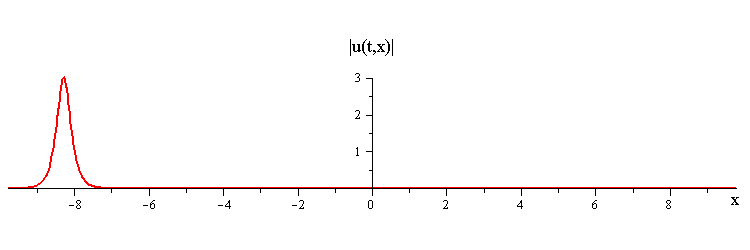### Colliding Travelling-Waves Solution

Collisions where a fast travelling wave with speed c1 and phase φ1 overtakes a slow travelling wave with speed c2 and phase φ2 are given by the special 2-soliton solution

u(t,x)= (c1c2)(c1 exp(iφ1) cosh(√c2 ξ2) + √c2 exp(iφ2) cosh(√c1 ξ1) )/( 4 √c1c2 cos(φ1 − φ2) + (√c1 − √c2)2 cosh(√c1 ξ1 + √c2 ξ2) + (√c1 + √c2)2 cosh(√c1 ξ1 − √c2 ξ2) )

where ξ1 = xc1t, ξ2 = xc2t are moving coordinates.

### Overlay of Travelling-Wave Solutions and Corresponding Collision Solution

Hirota merge-split interactionratio of fast to slow wave speeds is c1/c2 = 2.8
difference in phases is |φ1 − φ2| = 0.75 π

Hirota bounce-exchange interaction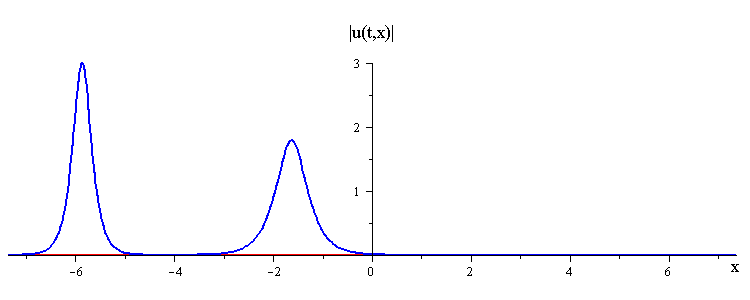ratio of fast to slow wave speeds is c1/c2 = 2.8
difference in phases is |φ1 − φ2| = 0.3 π

Hirota critical-phase interaction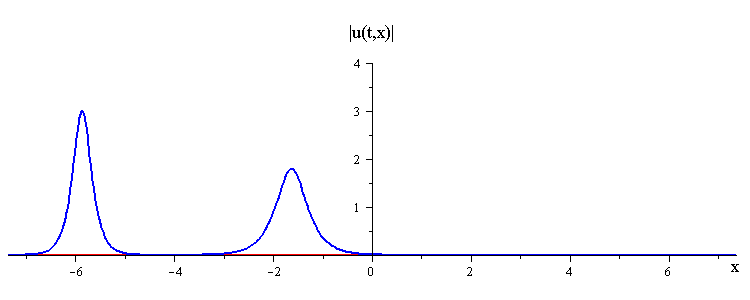ratio of fast to slow wave speeds is c1/c2 = 2.8
difference in phases is |φ1 − φ2| = 0.8689 π

Hirota absorb-emit interaction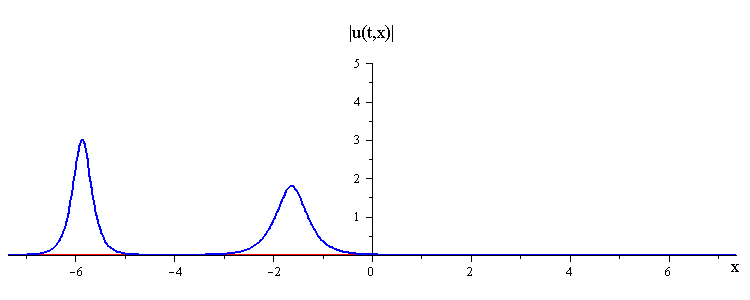ratio of fast to slow wave speeds is c1/c2 = 2.8
difference in phases is |φ1 − φ2| = 0.95 π

Hirota merge-split interaction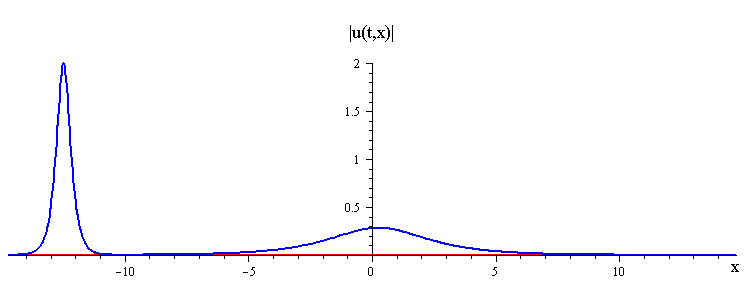ratio of fast to slow wave speeds is c1/c2 = 50
difference in phases is |φ1 − φ2| = 0.3 π
Hirota critical-phase interaction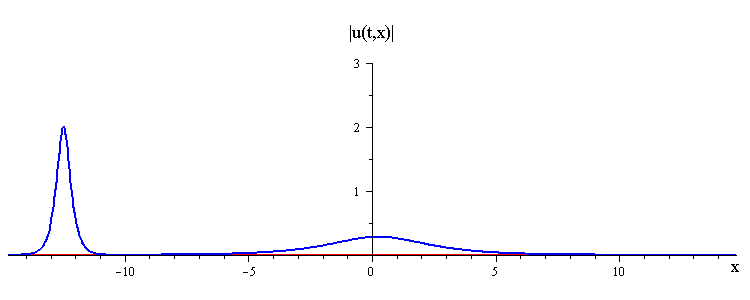ratio of fast to slow wave speeds is c1/c2 = 50
Hirota absorb-emit interaction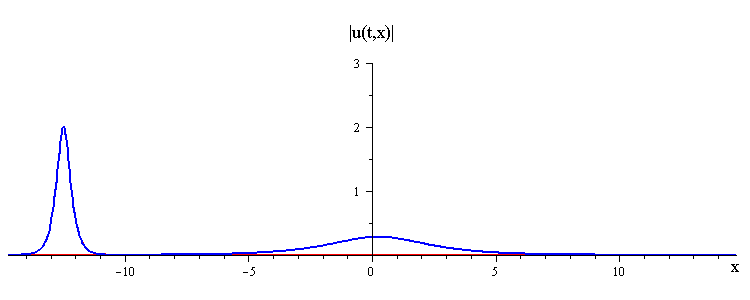ratio of fast to slow wave speeds is c1/c2 = 50